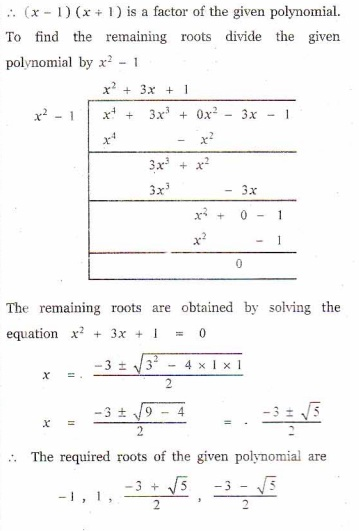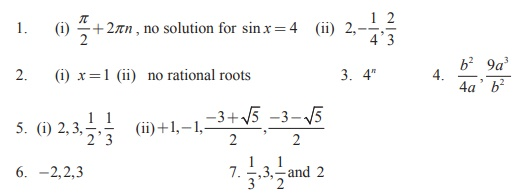Home | | Maths 12th Std | Exercise 3.5: Polynomial Equations with no Additional Information

# Exercise 3.5: Polynomial Equations with no Additional Information

Maths Book back answers and solution for Exercise questions - Mathematics : Theory of Equations: Polynomial Equations with no Additional Information: Exercise Questions with Answer, Solution

EXERCISE 3.5

1. Solve the following equations

(i) sin2- 5sin + 4 = 0

(ii) 12x3 + 8= 29x2 – 42. Examine for the rational roots of

(i) 2x3 - x2 -1 = 0

(ii) x8 - 3+1 = 0 .3. Solve : 8x 3/2n −8x-3/2n = 634. Solve :5. Solve the equations

(i)  6x4 - 35x3 + 62x2 - 35+ 6 = 0

(ii) x4 + 3x3 - 3-1 = 06. Find all real numbers satisfying  4- 3(2x+2 ) + 25 = 0 .7. Solve the equation 6x4 - 5x3 - 38x2 - 5+ 6 = 0 if it is known that 1/3 is a solution.